NCERT Solutions for Class 6 Maths Chapter 3 Playing with Numbers Ex 3.7

# NCERT Solutions for Class 6 Maths Chapter 3 Playing with Numbers Ex 3.7

## NCERT Solutions for Class 6 Maths Chapter 3 Playing with Numbers Ex 3.7

NCERT Solutions for Class 6 Maths Chapter 3 Playing with Numbers Ex 3.7 are the part of NCERT Solutions for Class 6 Maths. Here you can find the NCERT Solutions for Class 6 Maths Chapter 3 Playing with Numbers Ex 3.7.

### Ex 3.7 Class 6 Maths Question 1.

Renu purchases two bags of fertiliser of weights 75 kg and 69 kg. Find the maximum value of weight which can measure the weight of the fertiliser exact number of times.

Solution:
The maximum value of weight which can measure the weight of the fertiliser exact number of times = HCF of 75 kg and 69 kg
Prime factorisations of 75 and 69 are:Here, the common factor is 3.
HCF of 75 and 69 = 3
Hence, the maximum value of weight which can measure the weight of the fertiliser exact number of times is 3 kg.

### Ex 3.7 Class 6 Maths Question 2.

Three boys step off together from the same spot. Their steps measure 63 cm, 70 cm and 77 cm respectively. What is the minimum distance each should cover so that all can cover the distance in complete steps?

Solution:
The minimum distance that each boy should cover must be the least common multiple (LCM) of the measure of their steps.
To find the LCM of 63, 70 and 77, we can use division method.

LCM of 63, 70 and 77 = 2 × 3 × 3 × 5 × 7 × 11 = 6930
Hence, the required minimum distance is 6930 cm.

### Ex 3.7 Class 6 Maths Question 3.

The length, breadth and height of a room are 825 cm, 675 cm and 450 cm respectively. Find the longest tape which can measure the three dimensions of the room exactly.

Solution:
The longest tape required to measure the three dimensions of the room exactly = HCF of 825, 675 and 450
Prime factorisations of 825, 675 and 450 are:
825 = 3 × 5 × 5 × 11
675 = 3 × 3 × 3 × 5 × 5
450 = 2 × 3 × 3 × 5 × 5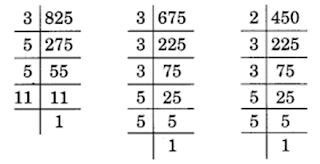Here, common factors are 3, 5, 5.
HCF of 825, 675 and 450 = 3 × 5 × 5 = 75
Hence, the length of the longest tape is 75 cm.

### Ex 3.7 Class 6 Maths Question 4.

Determine the smallest 3-digit number which is exactly divisible by 6, 8 and 12.

Solution:
The smallest 3-digit number is 100.
The smallest number divisible by 6, 8 and 12 = LCM of 6, 8 and 12

LCM of 6, 8 and 12 = 2 × 2 × 2 × 3 = 24

Thus, 24 is exactly divisible by 6, 8 and 12. But it is a 2-digit number.
Since, all the multiples of 24 will also be divisible by 6, 8 and 12.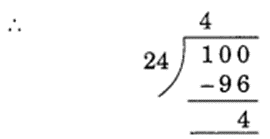So, the smallest multiple of 24 in three digits will be just above
100 = (100 – 4) + 24 = 96 + 24 = 120
Hence, the required smallest 3-digit number is 120.

### Ex 3.7 Class 6 Maths Question 5.

Determine the greatest 3-digit number exactly divisible by 8, 10 and 12.

Solution:
To find the LCM of 8, 10 and 12, we have

LCM of 8, 10 and 12 = 2 × 2 × 2 × 3 × 5 = 120
The greatest 3-digit number is 999.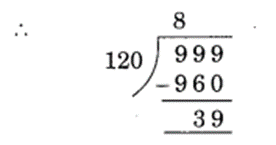Multiple of 120 just below 999 = 999 – 39 = 960
Hence, the required greatest 3-digit number is 960.

### Ex 3.7 Class 6 Maths Question 6.

The traffic lights at three different road crossings change after every 48 seconds, 72 seconds and 108 seconds respectively. If they change simultaneously at 7 a.m., at what time will they change simultaneously again?

Solution:

The time at which the traffic lights will change simultaneously again = LCM of 48, 72 and 108
To find the LCM of 48, 72 and 108, we have

LCM = 2 × 2 × 2 × 2 × 3 × 3 × 3 = 432
So, after 432 seconds, the traffic light will change simultaneously again.
Hence, the required time = 432 seconds = 7 minutes 12 seconds, i.e., 7 minutes 12 seconds past 7 a.m.

### Ex 3.7 Class 6 Maths Question 7.

Three tankers contain 403 litres, 434 litres and 465 litres of diesel respectively. Find the maximum capacity of a container that can measure the diesel of the three containers exact number of times.

Solution:
The maximum capacity of the required container = HCF of 403, 434 and 465
Prime factorisations of 403, 434 and 465 are:

The common factor is 31.
So, the HCF of 403, 434 and 465 = 31
Hence, the maximum capacity of the required container is 31 litres.

### Ex 3.7 Class 6 Maths Question 8.

Find the least number which when divided by 6, 15 and 18 leave remainder 5 in each case.

Solution:
The required number is 5 more than the LCM of 6, 15 and 18.

To find the LCM of 6, 15 and 18, we have

LCM of 6, 15 and 18 = 2 × 3 × 3 × 5 = 90
Here, 90 is the least number exactly divisible by 6, 15 and 18.
To get a remainder 5, the least number will be 90 + 5 = 95
Hence, the required number is 95.

### Ex 3.7 Class 6 Maths Question 9.

Find the smallest 4-digit number which is divisible by 18, 24 and 32.

Solution:
The smallest 4-digit number is 1000.
To find the LCM of 18, 24 and 32, we have

LCM = 2 × 2 × 2 × 2 × 2 × 3 × 3 = 288
Since, 288 is the smallest number which is exactly divisible by 18, 24 and 32.
But it is not a 4-digit number.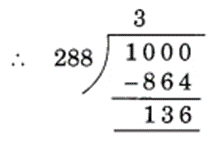So, the multiple of 288 just above 1000 is: 1000 – 136 + 288 = 1152
Hence, the required number is 1152.

### Ex 3.7 Class 6 Maths Question 10.

Find the LCM of the following numbers:
(a) 9 and 4
(b) 12 and 5
(c) 6 and 5
(d) 15 and 4
Observe a common property in the obtained LCMs. Is LCM the product of two numbers in each case?

Solution:
(a) To find the LCM of 9 and 4, we have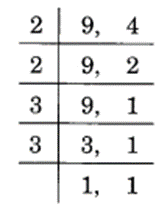LCM = 2 × 2 × 3 × 3 = 36
The product 9 and 4 = 9 × 4 = 36
Hence, the LCM of 9 and 4 = Product of 9 and 4

(b) To find LCM of 12 and 5, we have

LCM = 2 × 2 × 3 × 5 = 60
The product of 12 and 5 = 12 × 5 = 60
Hence, the LCM of 12 and 5 = Product of 12 and 5

(c) To find the LCM of 6 and 5, we have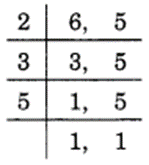LCM = 2 × 3 × 5 = 30
The product of 6 and 5 = 6 × 5 = 30
Hence, the LCM of 6 and 5 = Product of 6 and 5

(d) To find the LCM of 15 and 4, we have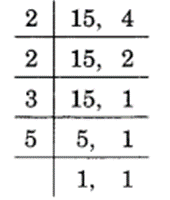LCM = 2 × 2 × 3 × 5 = 60
The product of 15 and 4 = 15 × 4 = 60
Hence, the LCM of 15 and 4 = Product of 15 and 4

### Ex 3.7 Class 6 Maths Question 11.

Find the LCM of the following numbers in which one number is the factor of the other.
(a) 5, 20
(b) 6, 18
(c) 12, 48
(d) 9, 45
What do you observe in the results obtained?

Solution:
(a) To find the LCM of 5 and 20, we have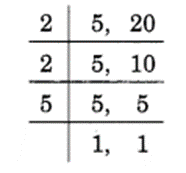LCM = 2 × 2 × 5 = 20
Hence, the LCM of 5 and 20 = 20

(b) To find the LCM of 6 and 18, we have

LCM = 2 × 3 × 3 = 18
Hence, the LCM of 6 and 18 = 18

(c) To find the LCM of 12 and 48, we have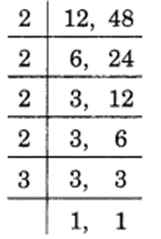LCM = 2 × 2 × 2 × 2 × 3 = 48
Hence, the LCM of 12 and 48 = 48

(d) To find the LCM of 9 and 45, we haveLCM = 3 × 3 × 5 = 45
Hence, the LCM of 9 and 45 = 45
From the above examples, we observe that if one number is a factor of the other number, then their LCM is the greater number.

You can also like these:

NCERT Solutions for Maths Class 7

NCERT Solutions for Maths Class 8

NCERT Solutions for Maths Class 9

NCERT Solutions for Maths Class 10

NCERT Solutions for Maths Class 11

NCERT Solutions for Maths Class 12